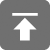# 工学1号馆

home

## Project Euler 7–10001st prime

Wu Yudong    September 26, 2017     欧拉计划   506

By listing the first six prime numbers: 2, 3, 5, 7, 11, and 13, we can see that the 6th prime is 13.

What is the 10 001st prime number?

#include <stdio.h>
#include <string.h>
#include <ctype.h>
#include <math.h>

int prim(int n)
{
int i;
for (i = 2; i * i <= n; i++) {
if (n % i == 0)
return 0;
}
return 1;
}

void solve(int n)
{
int i = 2;
int count = 0;
while (1) {
if (prim(i)) {
count++;
if (count == n)
break;
}
i++;
}
printf("%d\n", i);
}

int main()
{
int n = 10001;
solve(n);
return 0;
}